## MLP

In this tutorial, we will use Keras and MNIST dataset.

MNIST dataset is a opensource dataset for hand writing. Each image is in size 28 x 28 pixel.

Before we start get into Keras, let's prepare some visualize methods.

In :
``````import matplotlib.pyplot as plt
from IPython.display import Image

def show_images(images: list) -> None:
n: int = len(images)
f = plt.figure()
for i in range(n):
f.add_subplot(1, n, i + 1)
plt.imshow(images[i], cmap='gray')
plt.show(block=True)

def show_image(image) -> None:
plt.imshow(image, cmap='gray')
plt.show(block=True)

def show_online_image(target_url):
# Image(url= "https://gluon.mxnet.io/_images/dcgan.png")
Image(url= target_url)

def plot_images_labels_prediction(images,labels,prediction,idx,num=10):
fig=plt.gcf()
fig.set_size_inches(12, 14)
if num > 25: num=25
for i in range(0, num):
ax=plt.subplot(5, 5, i+1)
ax.imshow(images[idx], cmap='binary')
title="label=" + str(labels[idx])
if len(prediction) > 0:
title += ",predict=" + str(prediction[idx])
ax.set_title(title, fontsize=10)
ax.set_xticks([]);
ax.set_yticks([]);
idx += 1
plt.show()

def show_train_history(train_history):
fig=plt.gcf()
fig.set_size_inches(16, 6)
plt.subplot(121)
print(train_history.history.keys())

if "accuracy" in train_history.history.keys():
plt.plot(train_history.history["accuracy"])

if "val_accuracy" in train_history.history.keys():
plt.plot(train_history.history["val_accuracy"])

plt.title("Train History")
plt.xlabel("Epoch")
plt.ylabel("Accuracy")
plt.legend(["train", "validation"], loc="upper left")
plt.subplot(122)

if "loss" in train_history.history.keys():
plt.plot(train_history.history["loss"])

if "val_loss" in train_history.history.keys():
plt.plot(train_history.history["val_loss"])

plt.title("Train History")
plt.xlabel("Epoch")
plt.ylabel("Loss")
plt.legend(["train", "validation"], loc="upper left")
plt.show()
``````

#### Load and check your datas

You can load your dataset from Keras directly. Let's check how it looks like.

In :
``````from tensorflow import keras
from keras.datasets import mnist

(x_train, y_train), (x_test, y_test) = mnist.load_data()

# print out first 10 images in our training data
imgplot = show_images(x_train[0:10])

# check the size of our dataset
print("number of training images:",x_train.shape)
print("number of test images:",y_test.shape)``````
```Using TensorFlow backend. ```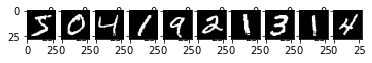```number of training images: 60000 number of test images: 10000 ```

Now we know the how many images we have in training data, and we know the structure of MLP.

How to make image into MLP input?
Simply, we make it into a vector.

In :
``````sample = x_train

# origin image
show_image(sample)

# input of MLP, a images were transfer into a vector
show_image(sample.reshape(1, 28 * 28))``````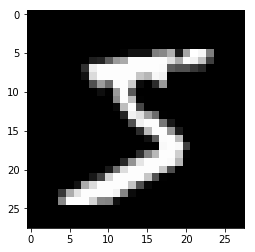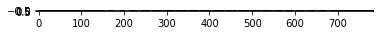In :
``````# update all the training, testing dataset.
x_train = x_train.reshape(60000, 28*28)
x_test = x_test.reshape(10000, 28*28)
x_train = x_train.astype('float32')
x_test = x_test.astype('float32')

# normalize them into range [0, 1]
x_train /= 255
x_test /= 255``````
In [ ]:
``````**Why Normalize need to divide by 255 for image?**

In computer vision, the color space were
R \[0,255\]
G \[0,255\]
B \[0,255\]

While we use gray images, the color space of R G B were same.
We can take color space \[3, High, Weight\] of an image as
[1, High, Weight].
Same as [H, W]  ``````

### Prepare your first model

In Keras, you can create model layers like a Queue.

In :
``````from tensorflow.keras import layers

num_classes = 10

# create a Sequential model
model = keras.Sequential(
[
# input layer. input data with size 28*28 and output size 256
# 256 means you set up your modul with 256 NN in this layer. This value is up to you.
layers.Dense(256, input_shape=(28*28,), activation='relu'),

# hidden layer. input data with size 256, which were same to output of input layer.
# output size 256, we set up 256 NN again in this hidden layer.
# no need to give input size here because keras already know.
layers.Dense(256, activation='relu'),

# output layer. the number of output should be your number of classification
layers.Dense(num_classes, activation='softmax')
]
);

# print out model structure
model.summary()
``````
```Model: "sequential_1" _________________________________________________________________ Layer (type) Output Shape Param # ================================================================= dense_3 (Dense) (None, 256) 200960 _________________________________________________________________ dense_4 (Dense) (None, 256) 65792 _________________________________________________________________ dense_5 (Dense) (None, 10) 2570 ================================================================= Total params: 269,322 Trainable params: 269,322 Non-trainable params: 0 _________________________________________________________________ ```

What we have in our model is:
input layer: input size = 2828+1, output size = 256. we have totally (2828+1)* 256 = 200960 parameters.
hidden layer: input size = 256 + 1, output size = 256. we have totally (256+1)* 256 = 65792 parameters.
output layer:input size = 256 + 1, output size = 10. we have totally (256+1)* 10 = 2570 parameters.

### Compile your model

We can now move on to compile our model.
Here are the parameters:

• optimizer : how to optimize your weights.
• loss : loss function.
• metrics : how to evaluate your model.

we don't disscuss how to select your optimizer and loss here. :)
Let's introduce 2 important setting in Machine Learning.

• Batch : there are 2 ways to update your weights in model

• update everytime after watching an input.
• update once after watching batch of inputs.

here we update once for 1000 inputs.

• Epochs : how many times you want to look overwhole your datas.

In :
``````model.compile(optimizer='adam',
loss='sparse_categorical_crossentropy',
metrics=['accuracy'])

batch_size = 256
epochs = 10
history = model.fit(x_train, y_train,
epochs=epochs,
validation_split=0.2)``````
```Train on 48000 samples, validate on 12000 samples Epoch 1/10 48000/48000 [==============================] - 6s 129us/sample - loss: 0.2197 - accuracy: 0.9340 - val_loss: 0.1113 - val_accuracy: 0.9671 Epoch 2/10 48000/48000 [==============================] - 6s 119us/sample - loss: 0.0888 - accuracy: 0.9728 - val_loss: 0.0903 - val_accuracy: 0.9742 Epoch 3/10 48000/48000 [==============================] - 6s 119us/sample - loss: 0.0626 - accuracy: 0.9797 - val_loss: 0.0935 - val_accuracy: 0.9731 Epoch 4/10 48000/48000 [==============================] - 6s 118us/sample - loss: 0.0467 - accuracy: 0.9851 - val_loss: 0.0906 - val_accuracy: 0.9749 Epoch 5/10 48000/48000 [==============================] - 6s 122us/sample - loss: 0.0351 - accuracy: 0.9888 - val_loss: 0.0929 - val_accuracy: 0.9764 Epoch 6/10 48000/48000 [==============================] - 7s 139us/sample - loss: 0.0291 - accuracy: 0.9905 - val_loss: 0.1253 - val_accuracy: 0.9694 Epoch 7/10 48000/48000 [==============================] - 6s 126us/sample - loss: 0.0240 - accuracy: 0.9916 - val_loss: 0.1389 - val_accuracy: 0.9701 Epoch 8/10 48000/48000 [==============================] - 6s 126us/sample - loss: 0.0215 - accuracy: 0.9931 - val_loss: 0.1377 - val_accuracy: 0.9694 Epoch 9/10 48000/48000 [==============================] - 6s 129us/sample - loss: 0.0207 - accuracy: 0.9932 - val_loss: 0.1080 - val_accuracy: 0.9775 Epoch 10/10 48000/48000 [==============================] - 6s 128us/sample - loss: 0.0182 - accuracy: 0.9943 - val_loss: 0.1445 - val_accuracy: 0.9706 ```

### Check result of model

How it works on testing data?

In :
``````scores, acc = model.evaluate(x_test, y_test, verbose=0)
print('Test loss:', scores)
print('Test accuracy:', acc)``````
```Test loss: 0.10330415646746806 Test accuracy: 0.9756 ```

It looks nice!

Let's visualize our result by printing out 25 images of label and predict result.

In :
``````prediction = model.predict_classes(x_test)
print('Test prediction:', prediction)

i = 0  # start from 0
j = 25 # end at 25

(_, _), (x_test_image, y_test_label) = mnist.load_data()
plot_images_labels_prediction(x_test_image,y_test_label,prediction,i,j)
``````
```Test prediction: [7 2 1 ... 4 5 6] ```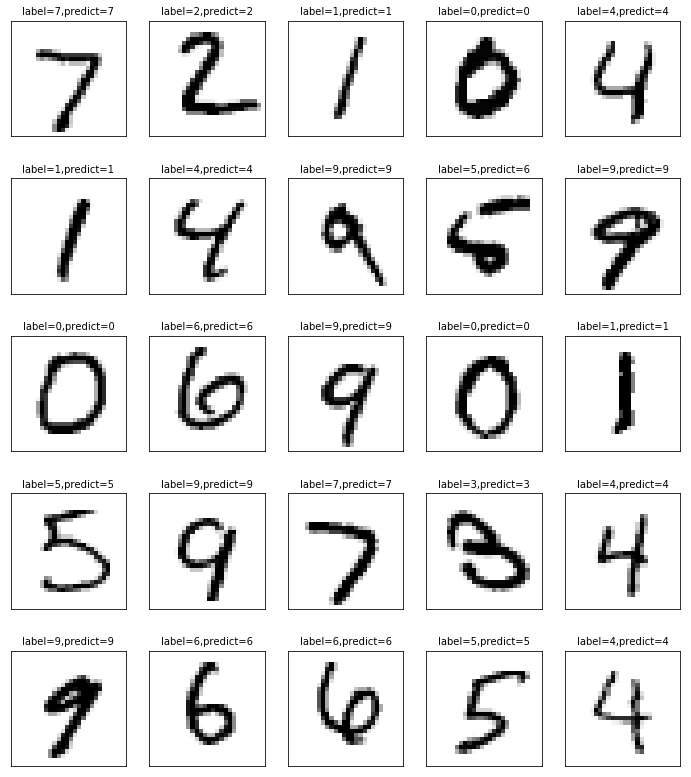In :
``````#show train history
show_train_history(history)
``````
```dict_keys(['loss', 'accuracy', 'val_loss', 'val_accuracy']) ```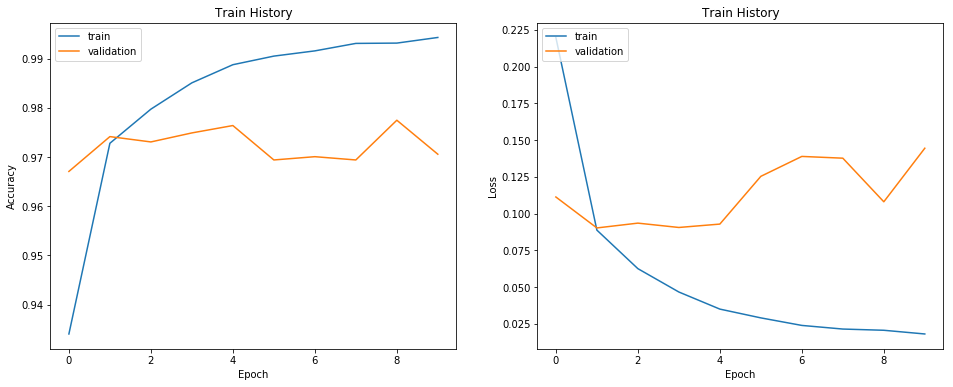In :
``````import pandas as pd

# create confusion matrix
pd.crosstab(y_test_label, prediction, rownames=['label'],colnames=['predict'])``````
Out:
In :
``````import jovian
jovian.commit()
``````
```[jovian] Saving notebook.. ```
```[jovian] Updating notebook "a3852a58642848449cc8fe84bb745c52" on https://jvn.io [jovian] Uploading notebook.. [jovian] Capturing environment.. [jovian] Committed successfully! https://jvn.io/littlenine/a3852a58642848449cc8fe84bb745c52 ```
```[jovian] Error: Failed to read Anaconda environment using command: "conda env export -n base --no-builds" ```